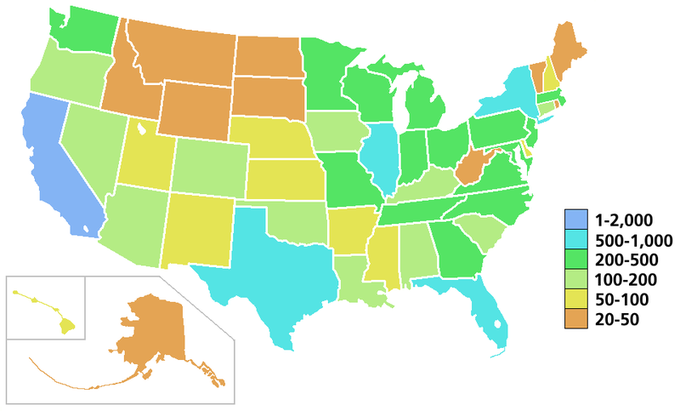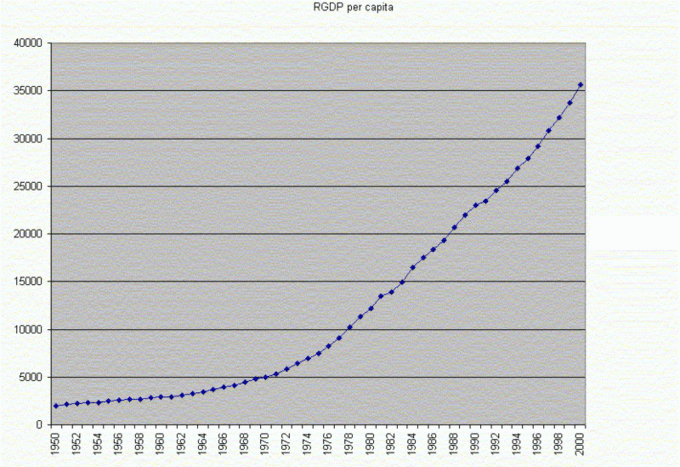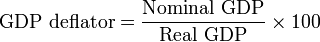## Calculating Real GDP

Real GDP growth is the value of all goods produced in a given year; nominal GDP is value of all the goods taking price changes into account.

### Learning Objectives

Calculate real and nominal GDP growth

### Key Takeaways

#### Key Points

• The following equation is used to calculate the GDP: GDP = C + I + G + (X – M) or GDP = private consumption + gross investment + government investment + government spending + (exports – imports).
• Nominal value changes due to shifts in quantity and price.
• In economics, real value is not influenced by changes in price, it is only impacted by changes in quantity. Real values measure the purchasing power net of any price changes over time.
• Real GDP accounts for inflation and deflation. It transforms the money-value measure, nominal GDP, into an index for quantity of total output.

#### Key Terms

• nominal: Without adjustment to remove the effects of inflation (in contrast to real).
• gross domestic product: Known also as GDP, this is a measure of the economic production of a particular territory in financial capital terms over a specific time period.

### Gross Domestic Product

The Gross domestic Product (GDP) is the market value of all final goods and services produced within a country in a given period of time. The GDP is the officially recognized totals. The following equation is used to calculate the GDP:

$GDP = C + I + G + (X - M)$

Written out, the equation for calculating GDP is:

GDP = private consumption + gross investment + government investment + government spending + (exports – imports).

For the gross domestic product, “gross” means that the GDP measures production regardless of the various uses to which the product can be put. Production can be used for immediate consumption, for investment into fixed assets or inventories, or for replacing fixed assets that have depreciated. “Domestic” means that the measurement of GDP contains only products from within its borders.

### Nominal GDP

The nominal GDP is the value of all the final goods and services that an economy produced during a given year. It is calculated by using the prices that are current in the year in which the output is produced. In economics, a nominal value is expressed in monetary terms. For example, a nominal value can change due to shifts in quantity and price. The nominal GDP takes into account all of the changes that occurred for all goods and services produced during a given year. If prices change from one period to the next and the output does not change, the nominal GDP would change even though the output remained constant.Nominal GDP: This image shows the nominal GDP for a given year in the United States.

### Real GDP

The real GDP is the total value of all of the final goods and services that an economy produces during a given year, accounting for inflation. It is calculated using the prices of a selected base year. To calculate Real GDP, you must determine how much GDP has been changed by inflation since the base year, and divide out the inflation each year. Real GDP, therefore, accounts for the fact that if prices change but output doesn’t, nominal GDP would change.Real GDP Growth: This graph shows the real GDP growth over a specific period of time.

In economics, real value is not influenced by changes in price, it is only impacted by changes in quantity. Real values measure the purchasing power net of any price changes over time. The real GDP determines the purchasing power net of price changes for a given year. Real GDP accounts for inflation and deflation. It transforms the money-value measure, nominal GDP, into an index for quantity of total output.

## The GDP Deflator

The GDP deflator is a price index that measures inflation or deflation in an economy by calculating a ratio of nominal GDP to real GDP.

### Learning Objectives

Explain how the calculation of the GDP deflator can measure inflation

### Key Takeaways

#### Key Points

• The GDP deflator is a measure of price inflation. It is calculated by dividing Nominal GDP by Real GDP and then multiplying by 100. (Based on the formula).
• Nominal GDP is the market value of goods and services produced in an economy, unadjusted for inflation. Real GDP is nominal GDP, adjusted for inflation to reflect changes in real output.
• Trends in the GDP deflator are similar to changes in the Consumer Price Index, which is a different way of measuring inflation.

#### Key Terms

• GDP deflator: A measure of the level of prices of all new, domestically produced, final goods and services in an economy. It is calculated by computing the ratio of nominal GDP to the real measure of GDP.
• real GDP: A macroeconomic measure of the value of the economy’s output adjusted for price changes (inflation or deflation).
• nominal GDP: A macroeconomic measure of the value of the economy’s output that is not adjusted for inflation.

The GDP deflator (implicit price deflator for GDP) is a measure of the level of prices of all new, domestically produced, final goods and services in an economy. It is a price index that measures price inflation or deflation, and is calculated using nominal GDP and real GDP.

### Nominal GDP versus Real GDP

Nominal GDP, or unadjusted GDP, is the market value of all final goods produced in a geographical region, usually a country. That market value depends on the quantities of goods and services produced and their respective prices. Therefore, if prices change from one period to the next but actual output does not, nominal GDP would also change even though output remained constant.

In contrast, real gross domestic product accounts for price changes that may have occurred due to inflation. In other words, real GDP is nominal GDP adjusted for inflation. If prices change from one period to the next but actual output does not, real GDP would be remain the same. Real GDP reflects changes in real production. If there is no inflation or deflation, nominal GDP will be the same as real GDP.

### Calculating the GDP Deflator

The GDP deflator is calculated by dividing nominal GDP by real GDP and multiplying by 100.GDP Deflator Equation: The GDP deflator measures price inflation in an economy. It is calculated by dividing nominal GDP by real GDP and multiplying by 100.

Consider a numeric example: if nominal GDP is $100,000, and real GDP is$45,000, then the GDP deflator will be 222 (GDP deflator = $100,000/$45,000 * 100 = 222.22).

In the U.S., GDP and GDP deflator are calculated by the U.S. Bureau of Economic Analysis.

### Relationship between GDP Deflator and CPI

Like the Consumer Price Index (CPI), the GDP deflator is a measure of price inflation/deflation with respect to a specific base year. Similar to the CPI, the GDP deflator of the base year itself is equal to 100. Unlike the CPI, the GDP deflator is not based on a fixed basket of goods and services; the “basket” for the GDP deflator is allowed to change from year to year with people’s consumption and investment patterns. However, trends in the GDP deflator will be similar to trends in the CPI.